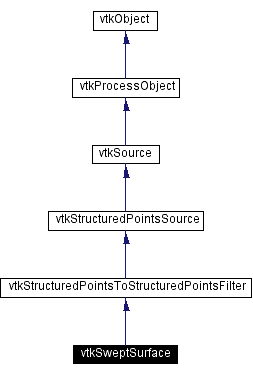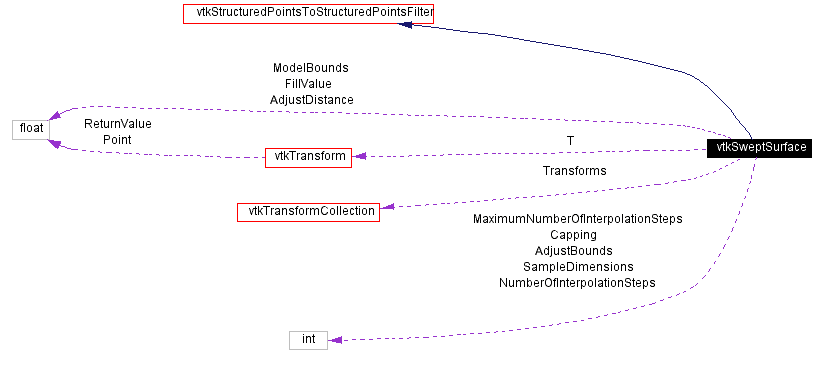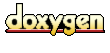Main Page   Class Hierarchy   Alphabetical List   Compound List   File List   Compound Members   File Members   Related Pages

# vtkSweptSurface Class Reference

given a path and input geometry generate an (implicit) representation of a swept surface. More...

`#include <vtkSweptSurface.h>`

Inheritance diagram for vtkSweptSurface:[legend]
Collaboration diagram for vtkSweptSurface:[legend]
List of all members.

## Public Methods

virtual const char * GetClassName ()
virtual int IsA (const char *type)
void PrintSelf (ostream &os, vtkIndent indent)
unsigned long int GetMTime ()
virtual void SetSampleDimensions (int, int, int)
virtual void SetSampleDimensions (int)
virtual int * GetSampleDimensions ()
virtual void GetSampleDimensions (int data)
virtual void SetTransforms (vtkTransformCollection *)
virtual vtkTransformCollectionGetTransforms ()
virtual void SetFillValue (float)
virtual float GetFillValue ()
virtual void SetNumberOfInterpolationSteps (int)
virtual int GetNumberOfInterpolationSteps ()
virtual void SetMaximumNumberOfInterpolationSteps (int)
virtual int GetMaximumNumberOfInterpolationSteps ()
virtual void SetCapping (int)
virtual int GetCapping ()
virtual void CappingOn ()
virtual void CappingOff ()
virtual void SetModelBounds (float data[])
virtual float * GetModelBounds ()
virtual void GetModelBounds (float data)
void SetModelBounds (float xmin, float xmax, float ymin, float ymax, float zmin, float zmax)

## Static Public Methods

vtkSweptSurface * New ()
int IsTypeOf (const char *type)
vtkSweptSurface * SafeDownCast (vtkObject *o)

## Protected Methods

vtkSweptSurface ()
~vtkSweptSurface ()
void Execute ()
void ExecuteInformation ()
void ComputeBounds (float origin, float ar, float bbox)
int ComputeNumberOfSteps (vtkTransform *t1, vtkTransform *t2, float bbox)
void SampleInput (vtkMatrix4x4 *m, int inDim, float inOrigin, float inAr, vtkDataArray *in, vtkDataArray *out)
void ComputeFootprint (vtkMatrix4x4 *m, int inDim, float inOrigin, float inSpacing, int Indicies)
void Cap (vtkDataArray *s)
void GetRelativePosition (vtkTransform &t, float *origin, float *position)
vtkMatrix4x4GetActorMatrixPointer (vtkTransform &t, float origin, float position, float orientation)
virtual void InterpolateStates (float *pos1, float *pos2, float *euler1, float *euler2, float t, float *posOut, float *eulerOut)

## Protected Attributes

int SampleDimensions 
float FillValue
float ModelBounds 
int NumberOfInterpolationSteps
int MaximumNumberOfInterpolationSteps
int Capping
vtkTransformCollectionTransforms

## Detailed Description

given a path and input geometry generate an (implicit) representation of a swept surface.

Date:
2001/12/17 15:42:38
Revision:
1.45.2.1

vtkSweptSurface is a filter that is used to create a surface defined by moving a part along a path. In this implementation, the path is defined as a list of transformation matrices (vtkTransform), and the part geometry is implicitly defined using a volume (i.e., distance scalars in structured point dataset). The input to the filter is the geometry (i.e., a structured point dataset) and the output is a structured point dataset (i.e., an implicit representation of the swept surface). If you wish to generate a polygonal representation of swept surface you will have to use a contouring filter (e.g., vtkContourFilter). (You may also wish to use vtkDecimate to reduce mesh size.)

The swept surface algorithm can be summarized as follows. A geometry (i.e. the input) is swept along a path (list of transforms). At each point on the path the input is re-sampled into a volume using a union operation. (Union means that the minimum scalar value is retained - minimum distance value for example.) At the end, an implicit representation of the swept surface is defined.

vtkImplicitModeller vtkContourFilter vtkDecimate
Tests:
vtkSweptSurface (Tests)

Definition at line 90 of file vtkSweptSurface.h.

## Constructor & Destructor Documentation

 vtkSweptSurface::vtkSweptSurface ( ) ` [protected]`

 vtkSweptSurface::~vtkSweptSurface ( ) ` [protected]`

## Member Function Documentation

 vtkSweptSurface* vtkSweptSurface::New ( ) ` [static]`
 Instantiate object with no start, end, or progress methods. Reimplemented from vtkStructuredPointsSource.

 virtual const char* vtkSweptSurface::GetClassName ( ) ` [virtual]`
 Return the class name as a string. This method is defined in all subclasses of vtkObject with the vtkTypeMacro found in vtkSetGet.h. Reimplemented from vtkStructuredPointsToStructuredPointsFilter.

 int vtkSweptSurface::IsTypeOf ( const char * type ) ` [static]`
 Return 1 if this class type is the same type of (or a subclass of) the named class. Returns 0 otherwise. This method works in combination with vtkTypeMacro found in vtkSetGet.h. Reimplemented from vtkStructuredPointsToStructuredPointsFilter.

 virtual int vtkSweptSurface::IsA ( const char * type ) ` [virtual]`
 Return 1 if this class is the same type of (or a subclass of) the named class. Returns 0 otherwise. This method works in combination with vtkTypeMacro found in vtkSetGet.h. Reimplemented from vtkStructuredPointsToStructuredPointsFilter.

 vtkSweptSurface* vtkSweptSurface::SafeDownCast ( vtkObject * o ) ` [static]`
 Will cast the supplied object to vtkObject* is this is a safe operation (i.e., a safe downcast); otherwise NULL is returned. This method is defined in all subclasses of vtkObject with the vtkTypeMacro found in vtkSetGet.h. Reimplemented from vtkStructuredPointsToStructuredPointsFilter.

 void vtkSweptSurface::PrintSelf ( ostream & os, vtkIndent indent ) ` [virtual]`
 Methods invoked by print to print information about the object including superclasses. Typically not called by the user (use Print() instead) but used in the hierarchical print process to combine the output of several classes. Reimplemented from vtkSource.

 virtual void vtkSweptSurface::SetSampleDimensions ( int , int , int ) ` [virtual]`
 Specify i-j-k dimensions to sample input with. The higher the resolution the lower the error but the greater the processing time.

 virtual void vtkSweptSurface::SetSampleDimensions ( int  ) ` [virtual]`
 Specify i-j-k dimensions to sample input with. The higher the resolution the lower the error but the greater the processing time.

 virtual int* vtkSweptSurface::GetSampleDimensions ( ) ` [virtual]`
 Specify i-j-k dimensions to sample input with. The higher the resolution the lower the error but the greater the processing time.

 virtual void vtkSweptSurface::GetSampleDimensions ( int data ) ` [virtual]`
 Specify i-j-k dimensions to sample input with. The higher the resolution the lower the error but the greater the processing time.

 virtual void vtkSweptSurface::SetTransforms ( vtkTransformCollection * ) ` [virtual]`
 Specify a path (i.e., list of transforms) that the input moves along. At least two transforms must be used to define a path.

 virtual vtkTransformCollection* vtkSweptSurface::GetTransforms ( ) ` [virtual]`
 Specify a path (i.e., list of transforms) that the input moves along. At least two transforms must be used to define a path.

 virtual void vtkSweptSurface::SetFillValue ( float ) ` [virtual]`
 Voxels are initialized to this value. By default a large floating point value is used, since the scalar values are assumed to be a distance function.

 virtual float vtkSweptSurface::GetFillValue ( ) ` [virtual]`
 Voxels are initialized to this value. By default a large floating point value is used, since the scalar values are assumed to be a distance function.

 virtual void vtkSweptSurface::SetNumberOfInterpolationSteps ( int ) ` [virtual]`
 Value specifies/controls interpolation between the nodes (i.e., transforms) defining the path. A positive value indicates the number of steps to take between transforms (i.e., interpolation is performed). A negative value indicates that no interpolation to be performed, that is, only the points defined at each transform are used (interpolation not performed). A zero value indicates that automatic interpolation is to be performed, that is, interpolation is computed so that potential errors fall below the error bounds defined in the text. By default, automatic computation is performed (Interpolation = 0).

 virtual int vtkSweptSurface::GetNumberOfInterpolationSteps ( ) ` [virtual]`
 Value specifies/controls interpolation between the nodes (i.e., transforms) defining the path. A positive value indicates the number of steps to take between transforms (i.e., interpolation is performed). A negative value indicates that no interpolation to be performed, that is, only the points defined at each transform are used (interpolation not performed). A zero value indicates that automatic interpolation is to be performed, that is, interpolation is computed so that potential errors fall below the error bounds defined in the text. By default, automatic computation is performed (Interpolation = 0).

 virtual void vtkSweptSurface::SetMaximumNumberOfInterpolationSteps ( int ) ` [virtual]`
 Set/get the maximum number of interpolation steps to take. This is useful if you are limited in computation time or just know that the number of computed steps should not exceed a certain value.

 virtual int vtkSweptSurface::GetMaximumNumberOfInterpolationSteps ( ) ` [virtual]`
 Set/get the maximum number of interpolation steps to take. This is useful if you are limited in computation time or just know that the number of computed steps should not exceed a certain value.

 virtual void vtkSweptSurface::SetCapping ( int ) ` [virtual]`
 The outer boundary of the sampling volume can be capped (i.e., assigned fill value). This will "close" the implicit model if the geometry approaches close to or passes through the boundary of the volume (i.e., defined by ModelBounds instance variable). Capping turns on/off this capability. By default capping is on.

 virtual int vtkSweptSurface::GetCapping ( ) ` [virtual]`
 The outer boundary of the sampling volume can be capped (i.e., assigned fill value). This will "close" the implicit model if the geometry approaches close to or passes through the boundary of the volume (i.e., defined by ModelBounds instance variable). Capping turns on/off this capability. By default capping is on.

 virtual void vtkSweptSurface::CappingOn ( ) ` [virtual]`
 The outer boundary of the sampling volume can be capped (i.e., assigned fill value). This will "close" the implicit model if the geometry approaches close to or passes through the boundary of the volume (i.e., defined by ModelBounds instance variable). Capping turns on/off this capability. By default capping is on.

 virtual void vtkSweptSurface::CappingOff ( ) ` [virtual]`
 The outer boundary of the sampling volume can be capped (i.e., assigned fill value). This will "close" the implicit model if the geometry approaches close to or passes through the boundary of the volume (i.e., defined by ModelBounds instance variable). Capping turns on/off this capability. By default capping is on.

 virtual void vtkSweptSurface::SetModelBounds ( float data[] ) ` [virtual]`
 Define the volume (in world coordinates) in which the sampling is to occur. Make sure that the volume is large enough to accommodate the motion of the geometry along the path. If the model bounds are set to all zero values, the model bounds will be computed automatically from the input geometry and path.

 virtual float* vtkSweptSurface::GetModelBounds ( ) ` [virtual]`
 Define the volume (in world coordinates) in which the sampling is to occur. Make sure that the volume is large enough to accommodate the motion of the geometry along the path. If the model bounds are set to all zero values, the model bounds will be computed automatically from the input geometry and path.

 virtual void vtkSweptSurface::GetModelBounds ( float data ) ` [virtual]`
 Define the volume (in world coordinates) in which the sampling is to occur. Make sure that the volume is large enough to accommodate the motion of the geometry along the path. If the model bounds are set to all zero values, the model bounds will be computed automatically from the input geometry and path.

 void vtkSweptSurface::SetModelBounds ( float xmin, float xmax, float ymin, float ymax, float zmin, float zmax )
 Define the volume (in world coordinates) in which the sampling is to occur. Make sure that the volume is large enough to accommodate the motion of the geometry along the path. If the model bounds are set to all zero values, the model bounds will be computed automatically from the input geometry and path.

 virtual void vtkSweptSurface::SetAdjustBounds ( int ) ` [virtual]`
 Control how the model bounds are computed. If the ivar AdjustBounds is set, then the bounds specified (or computed automatically) is modified by the fraction given by AdjustDistance. This means that the model bounds is expanded in each of the x-y-z directions.

 virtual int vtkSweptSurface::GetAdjustBounds ( ) ` [virtual]`
 Control how the model bounds are computed. If the ivar AdjustBounds is set, then the bounds specified (or computed automatically) is modified by the fraction given by AdjustDistance. This means that the model bounds is expanded in each of the x-y-z directions.

 virtual void vtkSweptSurface::AdjustBoundsOn ( ) ` [virtual]`
 Control how the model bounds are computed. If the ivar AdjustBounds is set, then the bounds specified (or computed automatically) is modified by the fraction given by AdjustDistance. This means that the model bounds is expanded in each of the x-y-z directions.

 virtual void vtkSweptSurface::AdjustBoundsOff ( ) ` [virtual]`
 Control how the model bounds are computed. If the ivar AdjustBounds is set, then the bounds specified (or computed automatically) is modified by the fraction given by AdjustDistance. This means that the model bounds is expanded in each of the x-y-z directions.

 virtual void vtkSweptSurface::SetAdjustDistance ( float ) ` [virtual]`
 Specify the amount to grow the model bounds (if the ivar AdjustBounds is set). The value is a fraction of the maximum length of the sides of the box specified by the model bounds.

 virtual float vtkSweptSurface::GetAdjustDistance ( ) ` [virtual]`
 Specify the amount to grow the model bounds (if the ivar AdjustBounds is set). The value is a fraction of the maximum length of the sides of the box specified by the model bounds.

 unsigned long int vtkSweptSurface::GetMTime ( ) ` [virtual]`
 Return this object's modified time. Reimplemented from vtkObject.

 void vtkSweptSurface::Execute ( ) ` [protected, virtual]`
 This method is the old style execute method Reimplemented from vtkSource.

 void vtkSweptSurface::ExecuteInformation ( ) ` [protected, virtual]`
 Reimplemented from vtkStructuredPointsToStructuredPointsFilter.

 void vtkSweptSurface::ComputeBounds ( float origin, float ar, float bbox ) ` [protected]`

 int vtkSweptSurface::ComputeNumberOfSteps ( vtkTransform * t1, vtkTransform * t2, float bbox ) ` [protected]`

 void vtkSweptSurface::SampleInput ( vtkMatrix4x4 * m, int inDim, float inOrigin, float inAr, vtkDataArray * in, vtkDataArray * out ) ` [protected]`

 void vtkSweptSurface::ComputeFootprint ( vtkMatrix4x4 * m, int inDim, float inOrigin, float inSpacing, int Indicies ) ` [protected]`

 void vtkSweptSurface::Cap ( vtkDataArray * s ) ` [protected]`

 void vtkSweptSurface::GetRelativePosition ( vtkTransform & t, float * origin, float * position ) ` [protected]`

 vtkMatrix4x4* vtkSweptSurface::GetActorMatrixPointer ( vtkTransform & t, float origin, float position, float orientation ) ` [protected]`

 virtual void vtkSweptSurface::InterpolateStates ( float * pos1, float * pos2, float * euler1, float * euler2, float t, float * posOut, float * eulerOut ) ` [protected, virtual]`

## Member Data Documentation

 int vtkSweptSurface::SampleDimensions` [protected]`
 Definition at line 208 of file vtkSweptSurface.h.

 float vtkSweptSurface::FillValue` [protected]`
 Definition at line 209 of file vtkSweptSurface.h.

 float vtkSweptSurface::ModelBounds` [protected]`
 Definition at line 210 of file vtkSweptSurface.h.

 int vtkSweptSurface::NumberOfInterpolationSteps` [protected]`
 Definition at line 211 of file vtkSweptSurface.h.

 int vtkSweptSurface::MaximumNumberOfInterpolationSteps` [protected]`
 Definition at line 212 of file vtkSweptSurface.h.

 int vtkSweptSurface::Capping` [protected]`
 Definition at line 213 of file vtkSweptSurface.h.

 int vtkSweptSurface::AdjustBounds` [protected]`
 Definition at line 214 of file vtkSweptSurface.h.

 float vtkSweptSurface::AdjustDistance` [protected]`
 Definition at line 215 of file vtkSweptSurface.h.

 vtkTransformCollection* vtkSweptSurface::Transforms` [protected]`
 Definition at line 217 of file vtkSweptSurface.h.

The documentation for this class was generated from the following file:

Generated on Thu Mar 28 14:43:29 2002 for VTK by1.2.11.1 written by Dimitri van Heesch, © 1997-2001